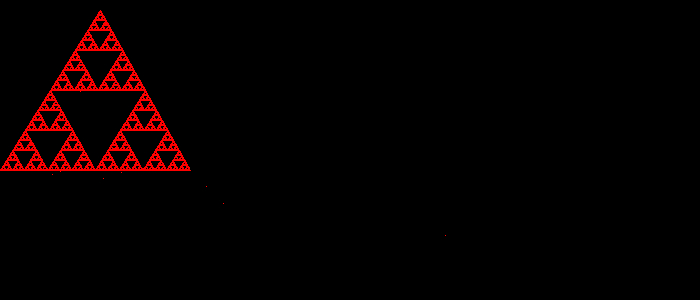# How to set a single pixel using imagesetpixel() function in PHP?

PHPServer Side ProgrammingProgramming

imagesetpixel() is an inbuilt function in PHP that is used to set a single pixel at the listed coordinate.

## Syntax

bool imagesetpixel(resource $image, int$x, int $y, int$color)

## Parameters

imagesetpixel() accepts four parameters: $image,$x, $y and$color.

• $image − Specifies the image resource to work on. •$x − Specifies the x-coordinate of the pixel.

• $y − Specifies the y-coordinate of the pixel. •$color − Specifies the color of the pixel.

### Return Values −

imagesetpixel() returns True on success and False on failure.

## Example 1

<?php
// Load the png image using imagecreatefromjpeg() function
$img = imagecreatefromjpeg('C:\xampp\htdocs\test\29.jpg'); // Draw the line using imagesetpixel() function$blue = imagecolorallocate($img, 255, 255, 0); for ($i = 0; $i < 1000;$i++) {
imagesetpixel($img,$i, 100, $blue); } // Show the output image to the browser header('Content-type: image/png'); imagepng($img);
?>

## Output## Example 2

<?php
$x = 700;$y = 300;
$gd = imagecreatetruecolor($x, $y);$corners = array('x' => 100, 'y' => 10);
$corners = array('x' => 0, 'y' => 170);$corners = array('x' => 190, 'y' => 170);
$blue = imagecolorallocate($gd, 255, 0, 0);
for ($i = 0;$i < 100000; $i++) { imagesetpixel($gd, round($x),round($y), $blue);$a = rand(0, 2);
$x = ($x + $corners[$a]['x']) / 2;
$y = ($y + $corners[$a]['y']) / 2;
}
?>